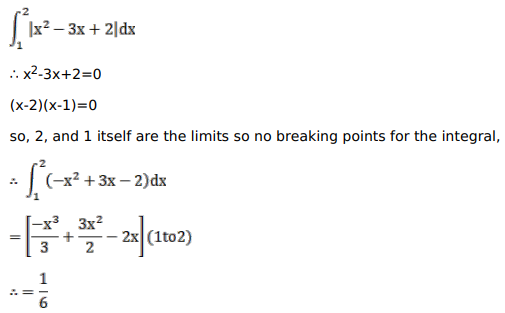# Mark against the correct answer in the following:`
Question:

Mark $(\sqrt{ })$ against the correct answer in the following:

$\int_{1}^{2}\left|x^{2}-3 x+2\right| d x=?$

A. $\frac{-1}{6}$

B. $\frac{1}{6}$

C. $\frac{1}{3}$

D. $\frac{2}{3}$

Solution: